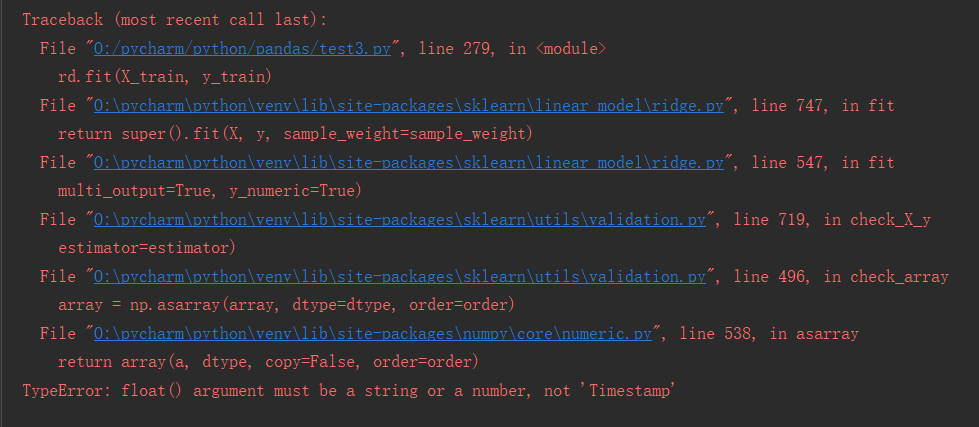•闪存
•博客
• 发言小组
• 投递新闻
• 提问博问
• 添加收藏
• 发布招聘
•文库

# 岭回归Ridge.fit(X_train, y_train)出现的问题

0
[待解决问题]# 划分训练机和测试集

X_train, X_test, y_train, y_test = train_test_split(X, y, test_size=0.2, random_state=1)
rd = Ridge(alpha=1)
rd.fit(X_train, y_train)0

您需要登录以后才能回答，未注册用户请先注册